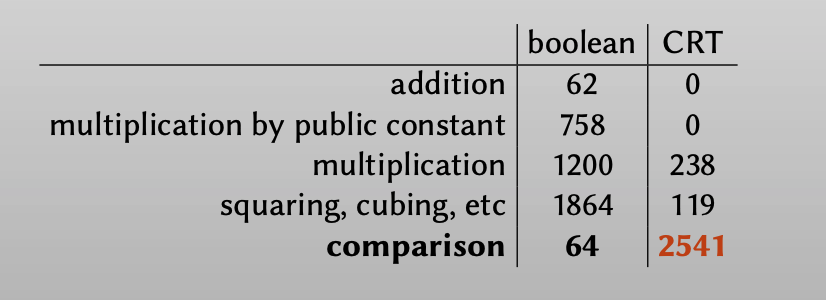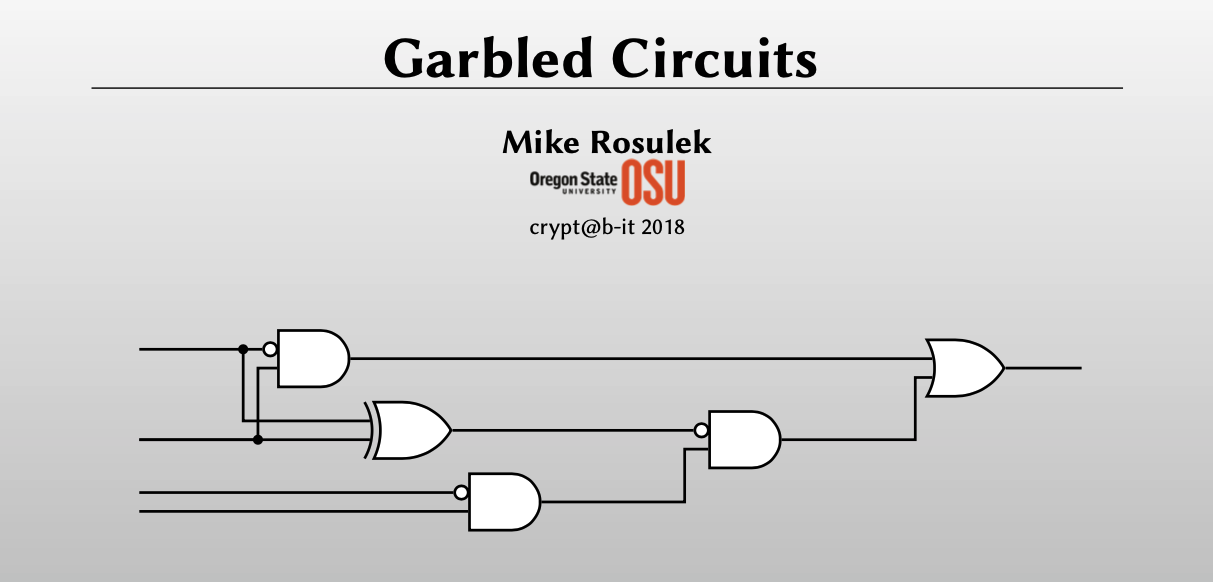# 「MPC-Mike Rosulek 」：Advanced Techniques and Optimizations for Garbled Circuits

1. Optimizations: How did garbled boolean circuits get so small?
2. New frontiers: How to garble arithmetic circuits?

# Optimizing garbled circuits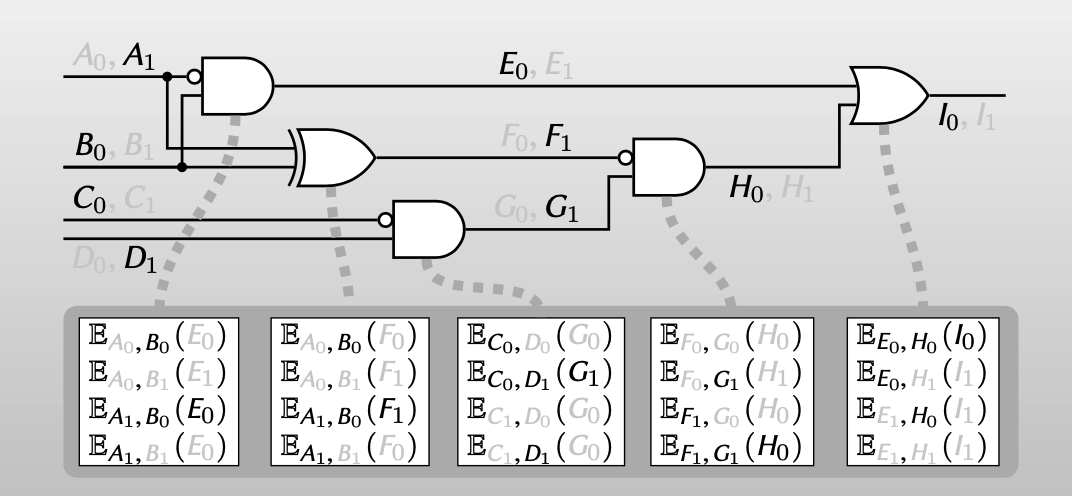Given garbled gate + one wire label per input wire:

• can learn only one output label (authenticity)
• cannot learn truth value of labels (privacy)

garbled circuits的计算性能和速度主要取决于他的大小（ciphertext的数量），但现实中garbled circuits的计算是非常快的。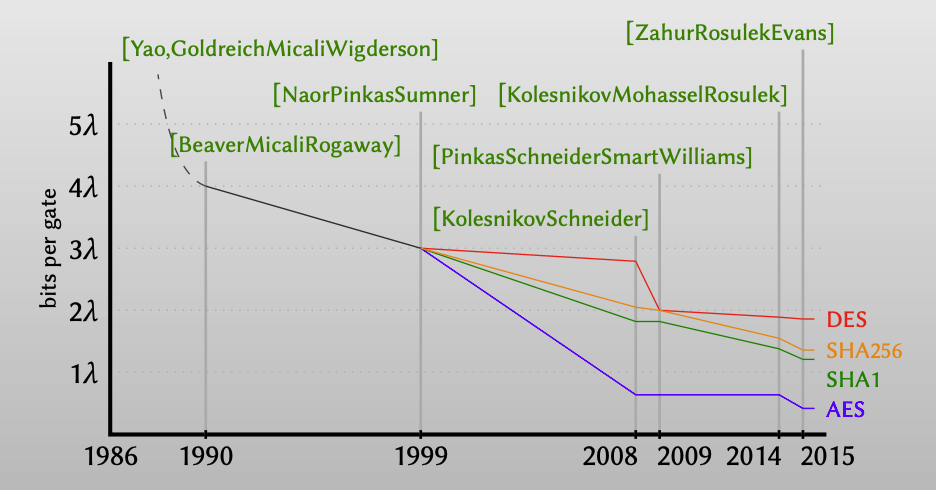## Ciphertext expansion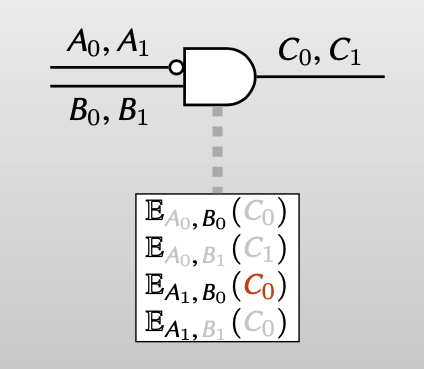## Point-and-permute

1. Assign color bits red & blue to wire labels.

2. Association between (red, blue ) <-> (True, Flase) is random for each wire.

【color bits 可以看作是一个bit mask，或者说是一个1-bit one-time pad。在为每一个wire的labels分配color bits时，是随机分配的：随机选择一个bit r (0或者1)，对于wire a，label的color bits的计算是 $a\oplus r$ 。】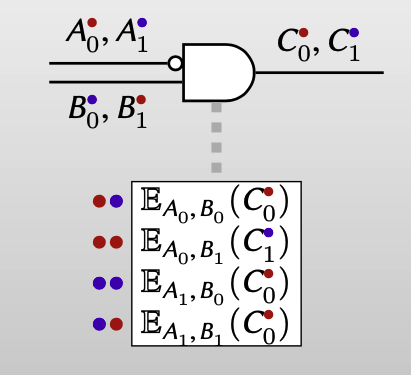3. A wire label reveals its own color(e.g. as last bit)

【可以在label后再附加一个bit作为color bit，来表示该wire label的颜色】

4. Order the 4 ciphertexts canonically, by color of keys.

【然后对表示garbled gate的4个ciphertexts按照指定的颜色组合排序】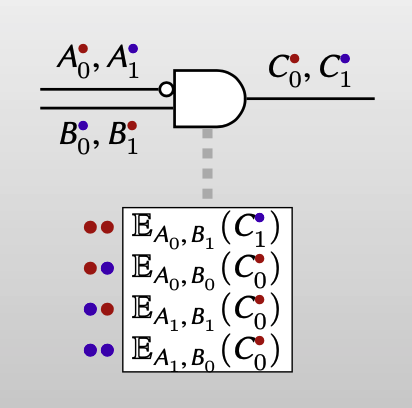5. Evaluate by decrypting ciphertext indexed by your colors

【这样evaluator在evaluate混淆电路时，就可以通过已知labels的颜色组合索引出正确的密文，再进行解密，不再需要对每一个密文进行尝试性解密。】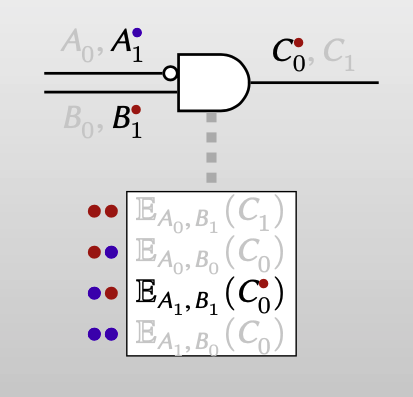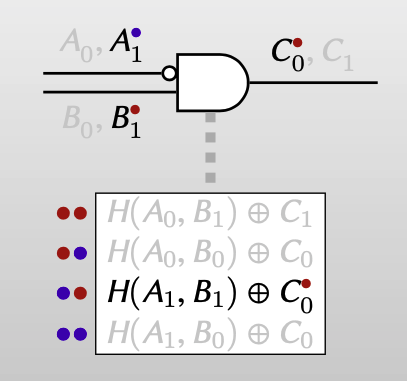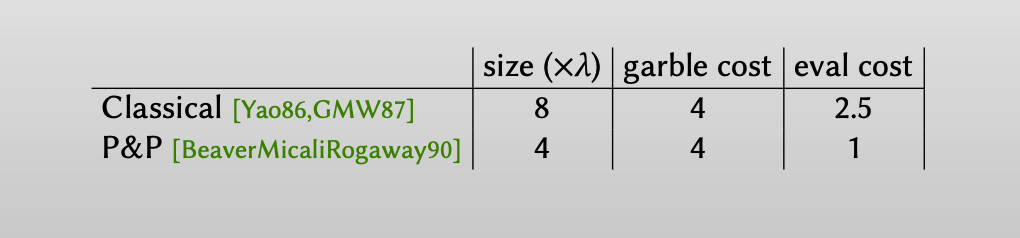• 假定单个label大小是 $\lambda$ ，garbled circuits的大小表示为ciphertexts的大小 。garble cost的值代表garbler加密时的开销，eval cost的值表示evaluator解密时的开销。
• 对于Yao的方案，因为有ciphertext expansion，所以garbled circuits的大小为 $8\lambda$ 。对每个garbled gate，都需要生成4个ciphertexts。而evaluator在评估电路时，每个ciphertext都有 1/4的概率是正确的，所以开销为 $2.5 = 1\times 1/4 + 2 \times 1/4 + 3\times 1/4 + 4\times 1/4$ .
• 对于Point-and-permute的方案，不需要ciphertext expansion，所以garbled circuits的大小为 $4\lambda$ 。在evaluator评估电路时，可以通过labels的颜色组合来索引密文，因此只需要1的开销。

## Garbled Row Reduction

Row Reduction[NaorPinkasSumner99] 是在Point-and-permute方案上进一步优化：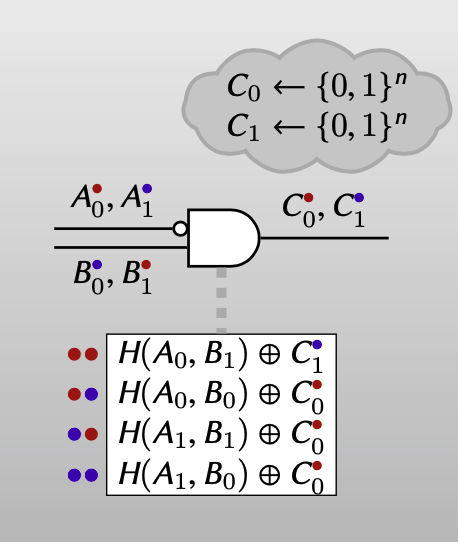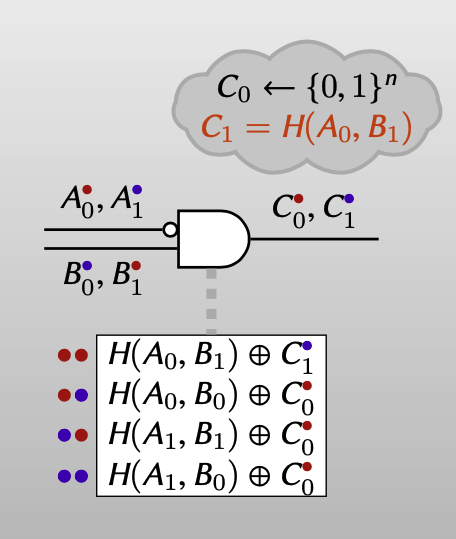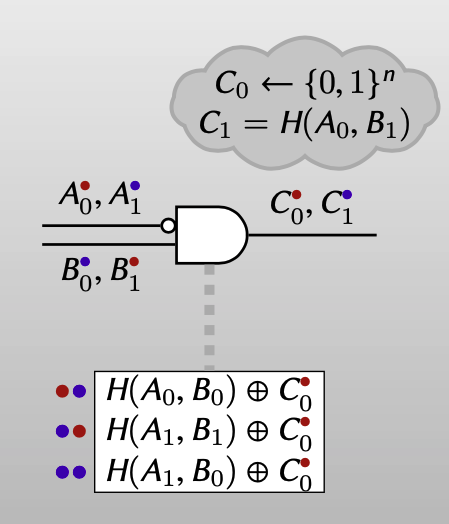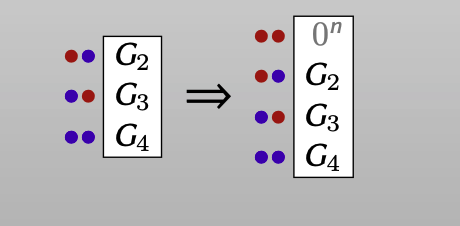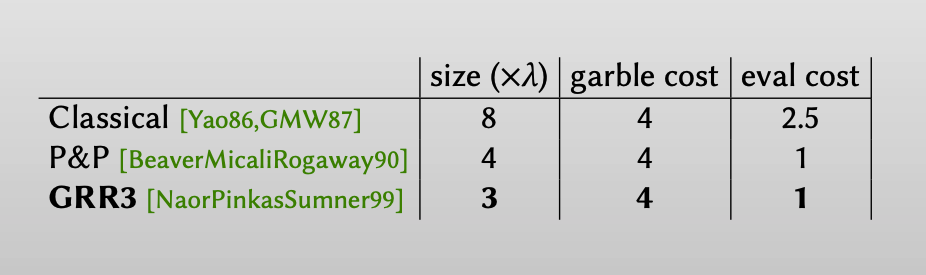GRR3方案相对于P&P方案，可以将garbled circuits的数量从4降为3。

## Free XOR

Free XOR[KolesnikovSchneider08] 是对异或门的优化技术。

1. Define offset of a wire ≡ XOR of its two labels

【Free XOR对wire定义了一种offset $\Delta$ ，该值其实等于两个labels的异或】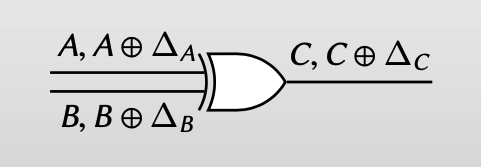2. Choose all wires in circuit to have same (secret) offset ∆

【Free XOR方案中，对所有的wires都选择了一个相同的保密的offset】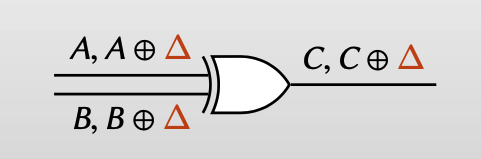3. Choose false output = false input ⊕ false input

【相较于之前方案的随机生成output wire的label，Free XOR让output wire的false output = false input ⊕ false input】

【也就是令 $C = A \oplus B$ (A: false , B: false, C: false)】

4. Evaluate by xoring input wire labels (no crypto)

【如此定义output wire的label，output wire的label可以通过input wire的label直接计算出来，而不需要再对其加密解密】

【false xor false = false ｜ $A \oplus B = C$ 】

【false xor true = true ｜ $A \oplus B \oplus \Delta = C\oplus \Delta$ 】

【true xor false = true ｜ $A \oplus \Delta \oplus B = C\oplus \Delta$ 】

【true xor true = false ｜ $A \oplus \Delta \oplus B \oplus \Delta = C$ 】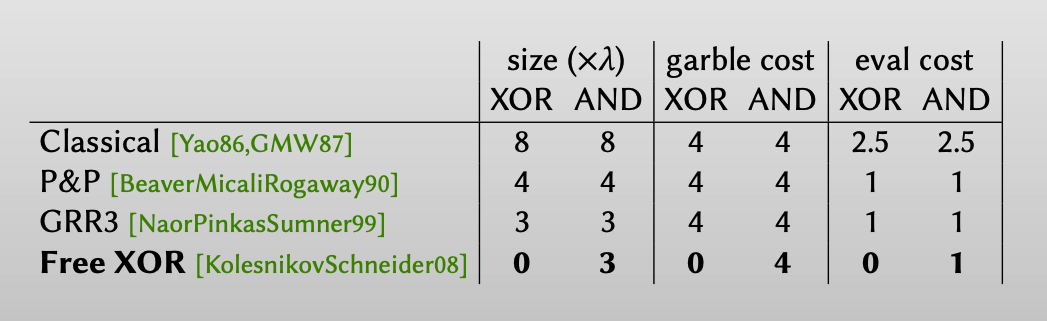## Row reduction $\times$ 2

Row reduction $\times$ 2[GueronLindellNofPinkas15] 方案是对Row Reduction方案的进一步优化，Row Reduction方案对输出wire的label选择上：$C_0$ 随机生成，$C_1$ 的值是能让P&P方案第一个ciphertext 为 $0^n$ 的方案，即 $C_1 = H(A_0, B_1)$ （式子只是针对下图的样例）。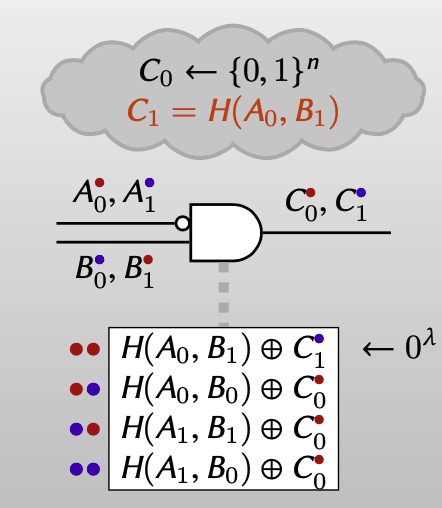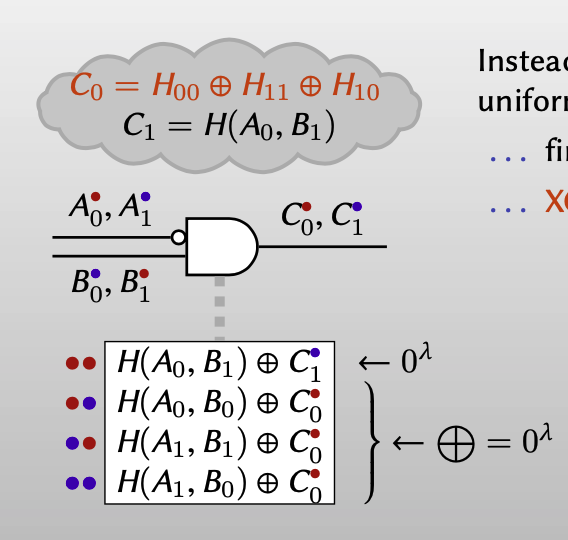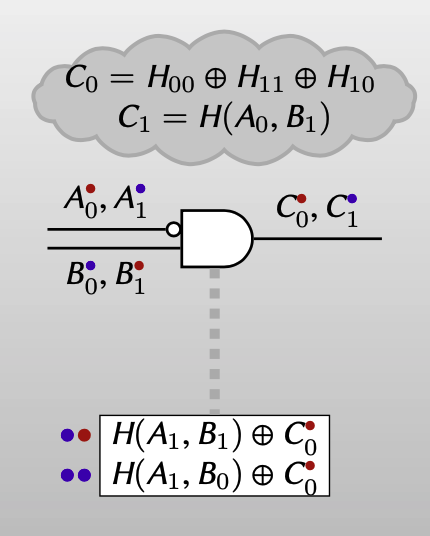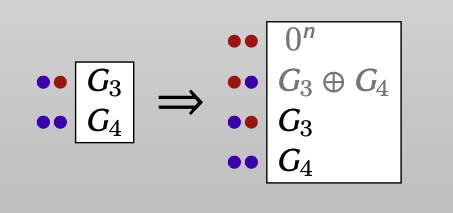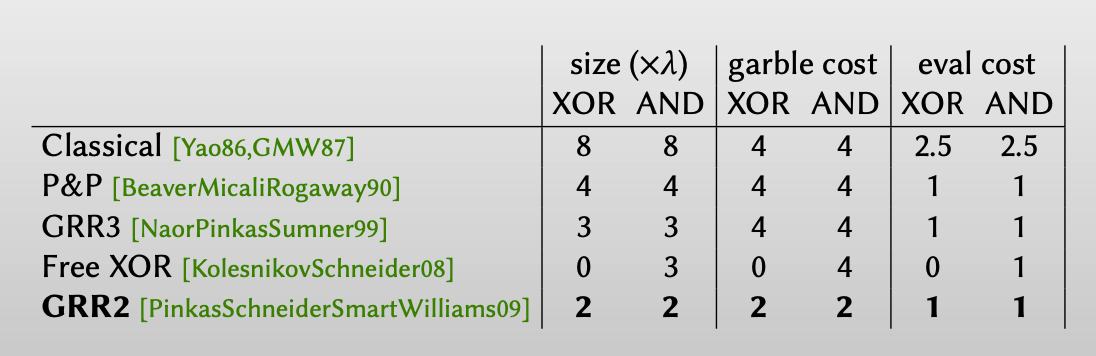## Half Gates

Half Gates 方案出自Samee Zahur, Mike Rosulek, David Evans的15年的文章：Two Halves Make a Whole: Reducing Data Transfer in Garbled Circuits using Half Gates. (Eurocrypt 2015)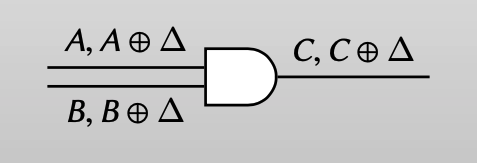### Garbler: half gate

• $a = 0$

如果$a = 0$, 这个门就变成一个一元门： $b \rightarrow 0$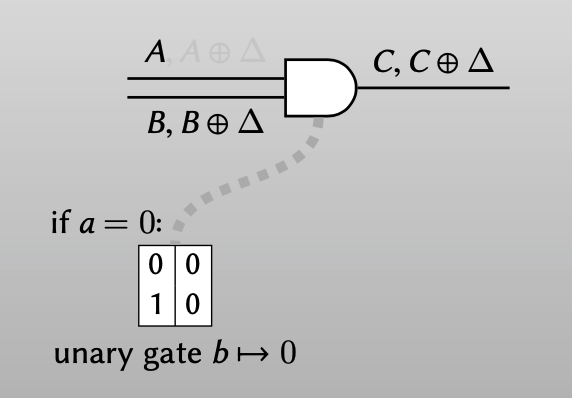可以用2个ciphertexts来表示这个门，evaluator解密后都得到output wire 的label $C$。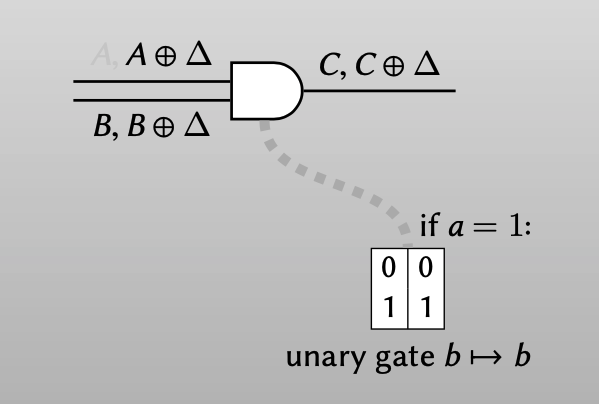• $a = 1$

如果$a = 1$, 这个门就变成一个一元门：$b\rightarrow b$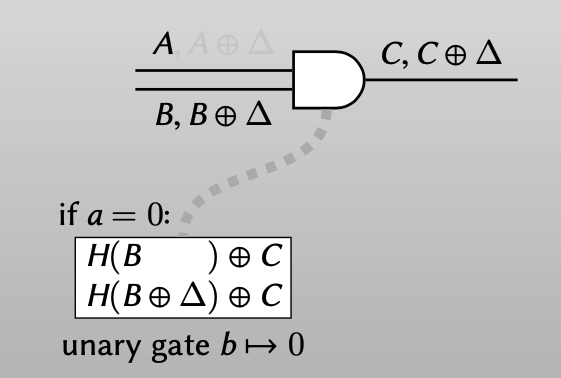同样可以用2个ciphertexts表示这个门，evaluator可以用label $B$解密得到output wire 的 label $C$，可以用 label$B\oplus \Delta$ 解密得到output wire 的label $C\oplus \Delta$ 。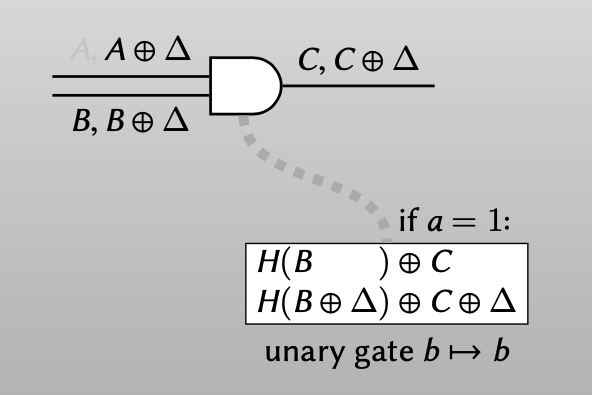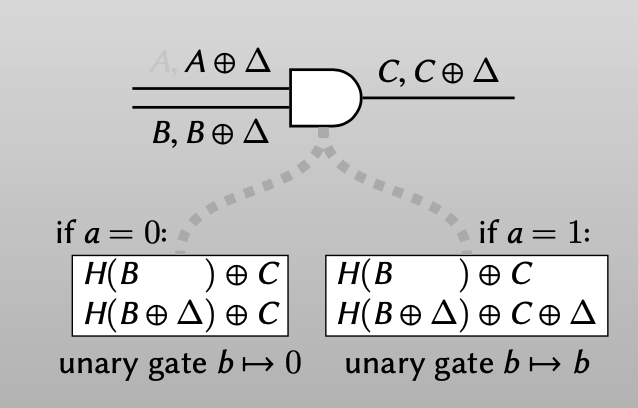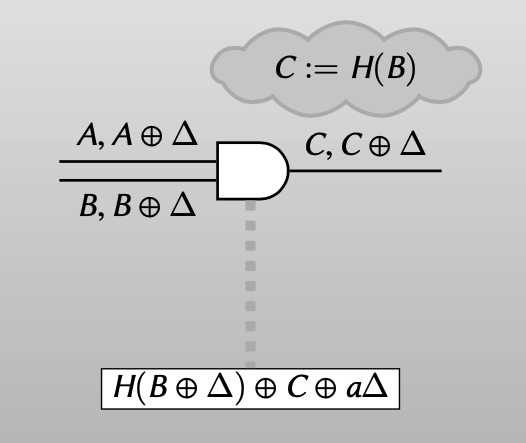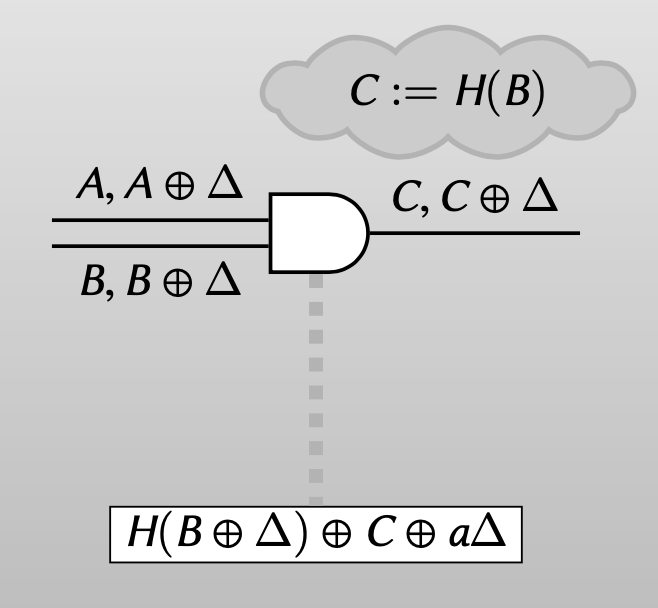### Evaluator: half gate

• Evaluator has B (knows label B is false): should obtain C (false)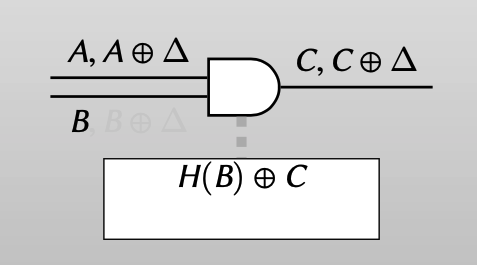• Evaluator has $B\oplus \Delta$ (knows label $B\oplus \Delta$ is true): should be able to transfer truth value from “a” wire to “c” wire.

【即如果evaluator知道wire b的label是true，那evaluate的结果的真值应该和wire a上label对应的真值相同（即使她不知道wire a上实际的真值是什么）】

Evaluator只要知道 $A\oplus C$ 的值，就可以实现 “transfer truth value from “a” wire to “c” wire.”

• false($A$) -> false ($C$) : $A\oplus A \oplus C = C$• true($A\oplus \Delta$ )-> true ($C\oplus \Delta$ ): $A\oplus \Delta \oplus A \oplus C = C\oplus \Delta$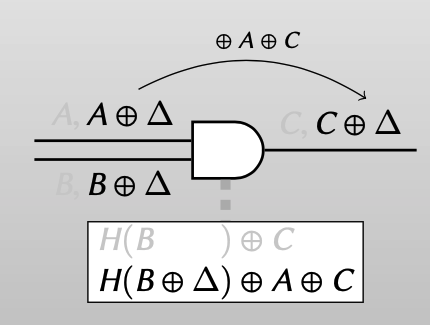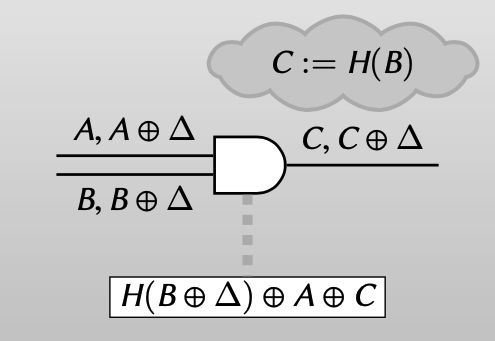### Two halves make a whole

• 对于garbler来说，garbler知道bit $r$的真值，true or false。
• 对于evaluator来说，他在evaluate这个AND gate时，他会得到wire a的label，虽然不知道这个label实际是对应着true还是false（即不知道 $a$ 的值），但是他知道这个label的color bit是什么（像P&P中提到的那样，可以把color bit放在label的最后一位），而这个color bit其实就是bit $a\oplus r$ 的真值，所以evaluator在evaluate时是知道 bit $a\oplus r$ 的真值的。

\begin{align} a\wedge b &= (a\oplus r \oplus r)\wedge b \ &=[(a\oplus r)\wedge b]\oplus [r\wedge b] \end{align}

• 对于左边的 $[(a\oplus r)\wedge b]$ ：evaluator知道 $a\oplus r$ 的真值，可以用上面提到的第二种half gate。
• 对于右边的 $[r\wedge b]$ : garbler知道 $r$ 的真值，可以用上面提到的第一种 half gate。
• 两个式子的xor运算：就可以使用Free-XOR 技术。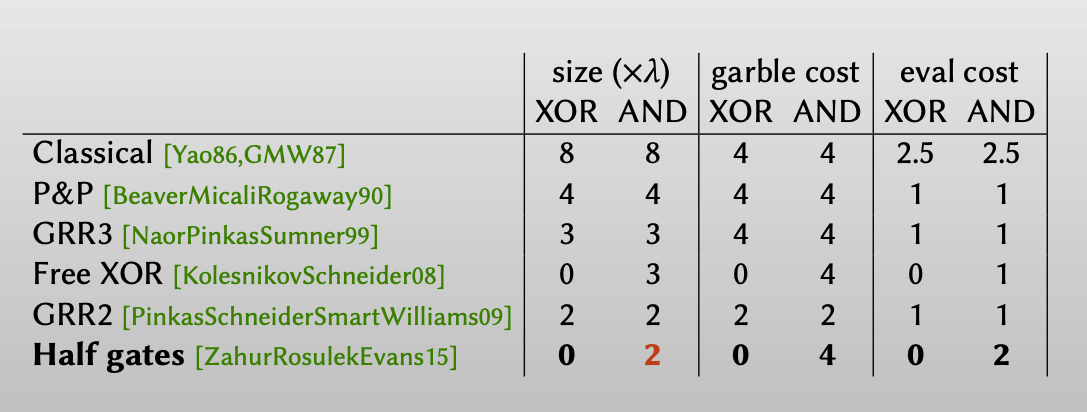# Garbling arithmetic circuits

## Generalized Free XOR

Free XOR Gerneralized Free XOR
Wire carries a truth value from ${0, 1}$ Wire carries a truth value from $\mathbb{Z}_m$
Wire labels are bit strings ${0, 1}^\lambda$ . Wire labels are tuples $(\mathbb{Z}_m)^\lambda$.
Global wire-label-offset $\Delta\in{0, 1}^\lambda$ Global wire-label-offset $\Delta\in(\mathbb{Z}_m)^\lambda$
false wire labe lis $A$ ；true wire label is $A\oplus \Delta$ Wire label encoding truth value $a\in \mathbb{Z}_m$ is $A+a\Delta$
⊕ is componentwise addition mod 2 + is componentwise addition mod m

Generalized Free XOR的核心就是：用wire label $A+a\Delta\in (\mathbb{Z}_m)^\lambda$ 来编码 $a\in \mathbb{Z}_m$ 。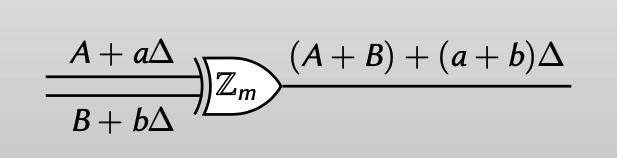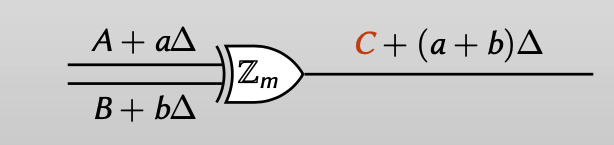## Garbling unary gates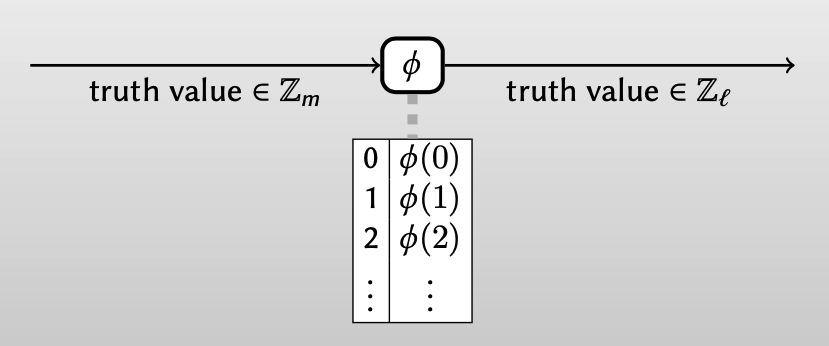Generalized Free XOR除了可以混淆模 $m$的加法电路，还可以混淆一元门。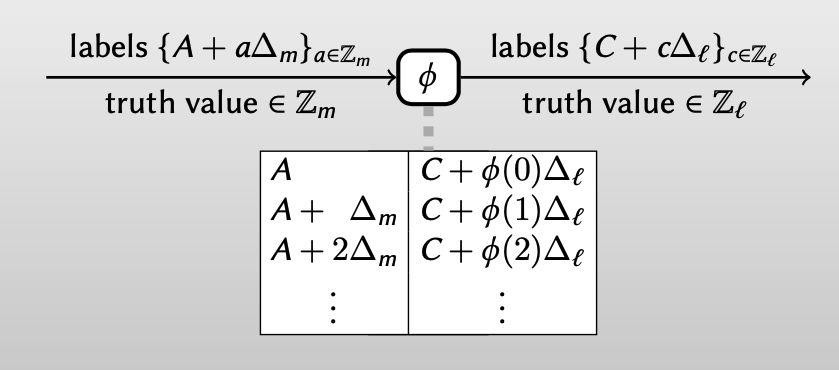## Generalized garbling tool

• Each wire has a preferred modulus $\mathbb{Z}_m$
• Wire-label-offset $\Delta_m$ global to all $\mathbb{Z}_m$ -wires
• Addition gates: all wires touching gate have same modulus
• Garbling cost: free
• Mult-by-constant gates: input/output wires have same modulus
• Garbling cost: free
• Unary gates: $\mathbb{Z}_m$ input and $\mathbb{Z}_l$ output
• Garbling cost: $m-1$ ciphertexts

## Arithmetic computations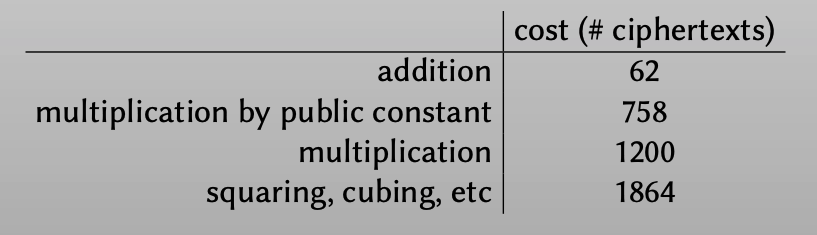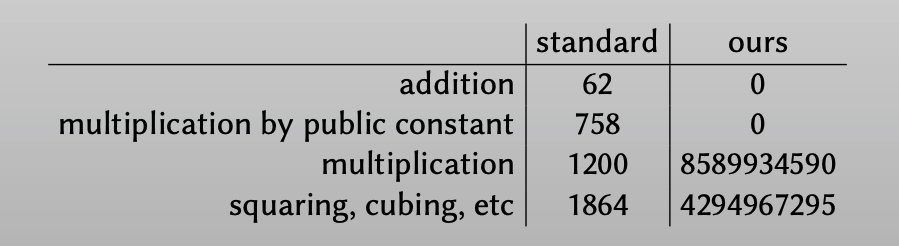## CRT form• Not so good:
• Converting from binary to CRT is not so good.
• Getting CRT valurs into the citcuit via OT is not so good.
• Kinda bad: (room for improvement)
• Comparing two CRT-encoded values
• Converting from CRT to binaty
• Integer division
• Modular reduction different than the CRT composite modulus (e.g., garbled RSA)

### Comparing CRT values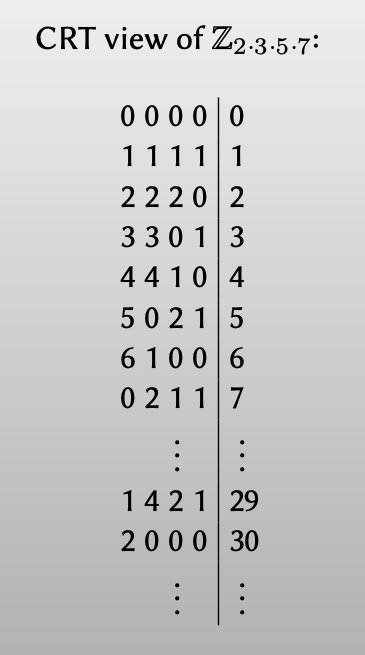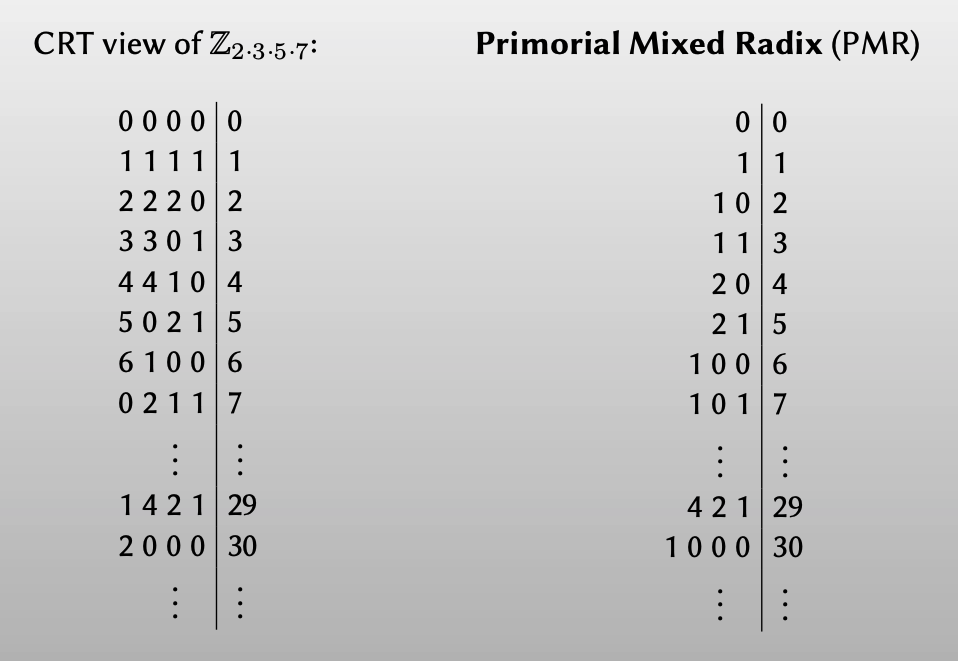1. Subtract x%3 − x%5 (mod 7 is fine)【相减】

• “Project” x%3 and x%5 to $\mathbb{Z}_7$ wires
2. Result has the same “constant segments” as what we want

• Apply unary projection：【再做一个一元映射】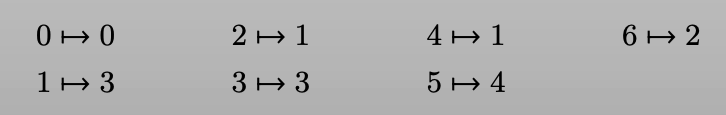• $(x\%p, x\%q)\mapsto \lfloor \frac{x}{p}\rfloor\%q$ 模块大约需要 $2p+2q$ 的开销。
• 而将CRT转换为PMR：对于每一个质数对都需要上述模块。

• 对于k-bit: CRT比较 $O(k^3)$